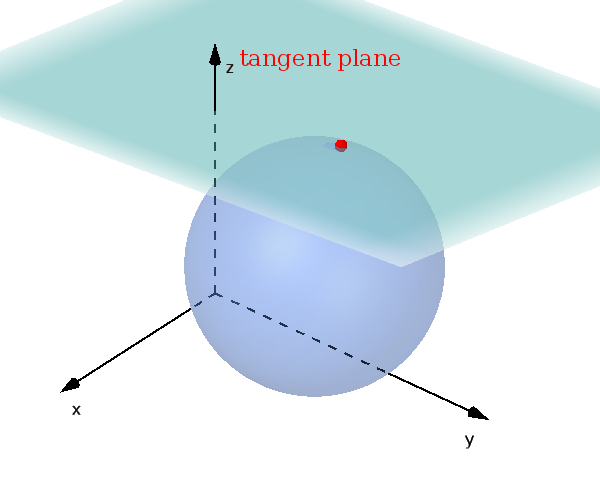Math Circles and spheres Tangent plane

# Tangent plane

A relative position between sphere and plane is the tangent plane.!

### Remember

If a plane and a sphere have exactly one common point, the plane is called a tangent plane and the point is called point of contact.

## Set up the tangent plane

If you have given the center $M$ of a sphere and the point of contact $B$, you can use the following formula to set up a tangent plane.

$\text{E: } (\vec{x}-\vec{OB})\cdot(\vec{OB}-\vec{OM})=0$

## Calculate point of contact

In the case of a tangent plane, the distance $d$ from the center of the sphere to the plane corresponds to the radius $r$ (see distance plane and point).

$r=d$

The point of contact $B$ can be calculated. To do this, set up the following equation of a line:

$g: \vec{x} = \vec{OM} + t \cdot \vec{n}$

$\vec{OM}$ is the center of the sphere and $\vec{n}$ is the normal vector of the plane.

The intersection of the line $g$ and plane $E$ is the point of contact.

i

### Method

1. Distance from $M$ to $E$
2. Check $r$ and $d$ (3 conditions, see above)
3. Set up $g$ and intersection point with $E$

### Example

$\text{E: } \left(\vec{x} - \begin{pmatrix} 5 \\4 \\ 3 \end{pmatrix}\right) \cdot \begin{pmatrix} 0\\ 0 \\ 1 \end{pmatrix}=0$

$k: (x+1)^2+(y-2)^2$ $+(z-1)^2=4$

1. #### Distance from $M$ to $E$

We can read the center of the sphere from the sphere equation.

$M(-1|2|1)$

We calculate the distance from the center $M$ to the plane $E$. First we set up the Hesse normal form.

$|\vec{n}|=\left|\begin{pmatrix} 0\\ 0 \\ 1 \end{pmatrix}\right|$ $=1$

Since the absolute value is 1, it is already a unit normal vector. We already have the Hesse normal form.

$\left(\vec{x} - \begin{pmatrix} 5 \\4 \\ 3 \end{pmatrix}\right) \cdot \begin{pmatrix} 0\\ 0 \\ 1 \end{pmatrix}=0$

The center point now only needs to be inserted for the distance calculation.

$d=\left|\left(\begin{pmatrix} -1 \\ 2 \\ 1 \end{pmatrix} - \begin{pmatrix} 5 \\4 \\ 3 \end{pmatrix}\right) \cdot \begin{pmatrix} 0\\ 0 \\ 1 \end{pmatrix}\right|$

$d=\left|\begin{pmatrix} -6 \\ 8 \\ -2 \end{pmatrix} \cdot \begin{pmatrix} 0\\ 0 \\ 1 \end{pmatrix}\right|$

$d=\left|0+0-2\right|$ $=|-2|$ $=2$

2. #### Check condition

First you read the radius from the sphere equation.

$r=\sqrt{4}=2$

We now look at which of the three cases is present.

$2=2$

$d=r$

The distance is equal to the radius ($d=r$), the plane touches the sphere and there is therefore a point of contact.

3. #### Set up $g$ and intersection point with $E$

Now it's about calculating the new point of contact. An equation of a line is set up as described above.

$g: \vec{x} = \vec{OM} + t \cdot \vec{n}$

$g: \vec{x} = \begin{pmatrix} -1 \\ 2 \\ 1 \end{pmatrix} + t \cdot \begin{pmatrix} 0\\ 0 \\ 1 \end{pmatrix}$

The intersection point of the line and the plane corresponds to the point of contact $B$.

The straight line equation is inserted for $\vec{x}$ in the plane.

$(\begin{pmatrix} -1 \\ 2 \\ 1 \end{pmatrix}$ $+ t \cdot \begin{pmatrix} 0\\ 0 \\ 1 \end{pmatrix}$ $- \begin{pmatrix} 5 \\4 \\ 3 \end{pmatrix}) \cdot \begin{pmatrix} 0\\ 0 \\ 1 \end{pmatrix}=0$

$\begin{pmatrix} -6 \\ -2 \\ t-2 \end{pmatrix} \cdot \begin{pmatrix} 0\\ 0 \\ 1 \end{pmatrix}=0$

$0+0+1\cdot(t-2)=0$
$t-2=0\quad|+2$
$t=2$

The point of contact $B$ is obtained by inserting the calculated $t$ into the equation of a line.

$\vec{OB} = \begin{pmatrix} -1 \\ 2 \\ 1 \end{pmatrix} + 2 \cdot \begin{pmatrix} 0\\ 0 \\ 1 \end{pmatrix}$ $=\begin{pmatrix} -1 \\ 2 \\ 3 \end{pmatrix}$

$B(-1|2|3)$